Vectors

Scalars are quantities that only have a magnitude like mass, speed, and electric field strength. Many times it is often useful to have a quantity that has not only a magnitude but also a direction; such a quantity is called a vector. Examples of quantities represented by vectors include velocity, acceleration, and virtually any type of force (frictional, gravitational, electric, magnetic, etc.). Note that all of these quantities not only have a magnitude (such as speed - the madnitude of the velocity vector) but also occur or act in a given direction.

As an example of when vectors are necessary, suppose a plane traveling at 300 mph to the north with no wind present encounters a westerly crosswind of 50 mph. The resultant velocity of the plane is the sum of the velocities of the wind and the plane. To find this resultant velocity, we must use vectors.

We represent vectors with ordered pairs in pointed brackets to distinguish them from ordered pairs in normal parentheses which represent points. The vector <1,4> is a two-dimensional vector, or directed line segment, from any point (x,y) to the point (x+1,y+4). Likewise, the vector <a,b,c> is a three-dimensional vector from any point (x,y,z) to the point (x+a,y+b,z+c). It is important to remember that a vector is independent of its position in the coordinate system.

The magnitude (or length) of a vector v with initial point (x_1,y_1,z_1) and terminal point (x_2,y_2,z_2) is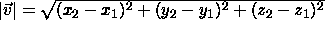Vectors obey the natural intuitive laws of addition and scalar multiplication: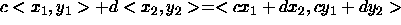The figures below illustrate the operations of addition and scalar multiplication in the two-dimensional case.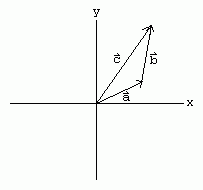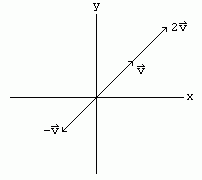The vectors i=<1,0,0>, j=<0,1,0>, and k=<0,0,1> are special since the have unit length and point in the directions of the x-, y-, and z-axes. Any vector in three dimensions can be represented as a linear combination of these three vectors:

<x,y,z>=x<1,0,0>+y<0,1,0>+z<0,0,1>

The x-, y-, and z-components of a vector are the vectors x<1,0,0>, y<0,1,0>, and z<0,0,1>, respectively.

#### Example

The vector from the origin to a point P=(x,y,z) has a special name. It is called the position vector of the point since it describes the position of the point relative to the origin.

#### Example

To solve the example given above involving the plane, we define the direction <1,0> to be east and the direction <0,1> to be north and thus represent the velocity of the plane by p=<300,0> and the velocity of the wind by w=<0,-50>. The resultant velocity of the plane is the sum of these two vectors: r=<300,0>+<0,-50>=<300,-50>. Thus, the plane actually travels in a direction to the west of north.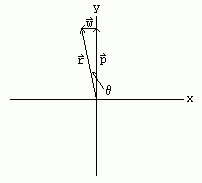The vertical component of this resultant vector is 300<0,1> and the horizontal component is -50<1,0>. Using the figure above we see that tan(theta)=-50/300=-1/6 which implies theta=-9.5 degrees. Thus, using the diagram as a guide, we see that the resulting motion of the plane is 9.5 degrees west of north.

Vectors can of course be represented in other coordinate systems. For the spherical coordinate system, instead of the components of a vector being in the x-, y-, and z-directions, the components would be in the rho-, theta-, and phi-directions. <1,0,0> would be the unit vector in the rho-direction. Although the formula for the magnitude of a vector is much simpler since it is just the magnitude of the rho-component..